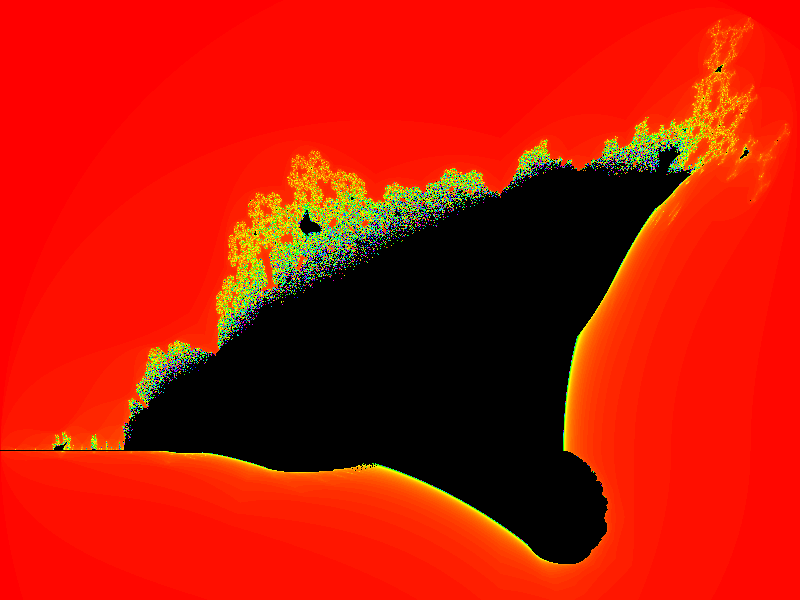# Burning ship fractal with generativepy

Martin McBride, 2020-10-28
Tags burning ship fractal
Categories generativepy generative art

generativepy has moved to the pythoninformer.com website, and this page might not be up to date. Please visit the new location.

The burning ship fractal is another famous fractal that can be implemented easily with generativepy.

Like the Mandelbrot set, the burning ship is an escape-time fractal.

## Burning ship formula

The burning ship equations are similar to the Mandelbrot equations:

xnext = x*x - y*y + c1
ynext = 2*abs(x*y) + c2


Where c1 and c2 are two constant values.

The difference compared to the Mandelbrot equations in that the ynext equation uses abs(x*y) rather than just x*y. The absolute value is the positive size of the number, ignoring it's sign. So abs(3) is 3, abs(-3) is also 3.

Here is the image created:## The full code

Here is the full code for the burning ship fractal:

from generativepy.bitmap import make_bitmap, Scaler
from generativepy.color import Color

MAX_COUNT = 200
BLACK = Color(0)

def calc(c1, c2):
x = y = 0
for i in range(MAX_COUNT):
x, y = x*x - y*y + c1, abs(2*x*y) + c2
if x*x + y*y > 4:
return i
return -1

def build_color_table(size):
table = [Color.of_hsl(i/size, 1, 0.5) for i in range(size)]
return table

def paint(image, pixel_width, pixel_height, frame_no, frame_count):
scaler = Scaler(pixel_width, pixel_height, width=3.2, startx=-2, starty=-1.8)

colors = build_color_table(MAX_COUNT)

for px in range(pixel_width):
for py in range(pixel_height):
x, y = scaler.device_to_user(px, py)
count = calc(x, y)
col = BLACK if count < 0 else colors[count]
image.putpixel((px, py), col.as_rgb_bytes())

make_bitmap('burning-ship.png', paint, 800, 600)


As well as changing the formula we have also slighlty adjusted the area of the user space (in the call to Scaler), to better fit the image.

Copyright (c) Axlesoft Ltd 2021